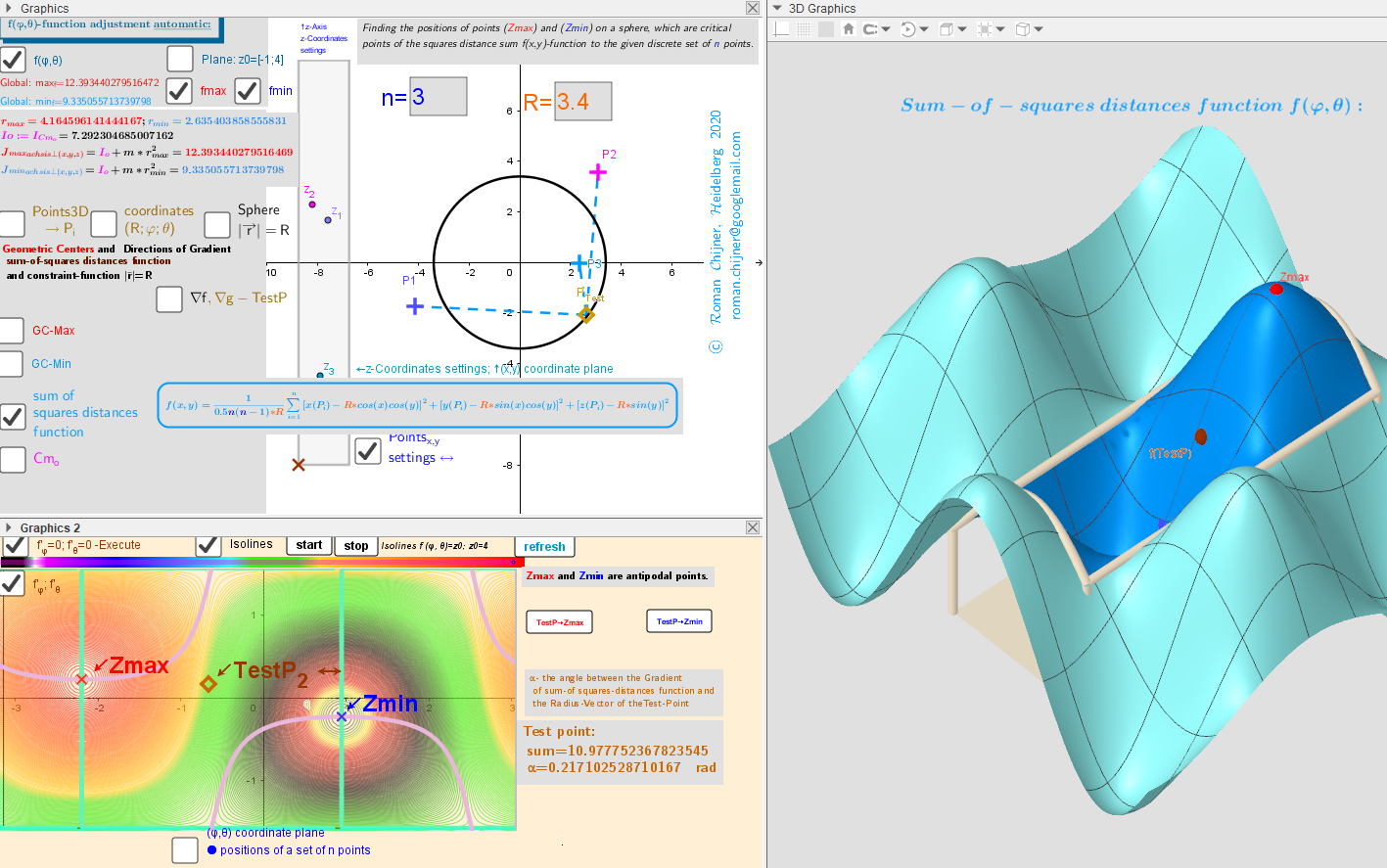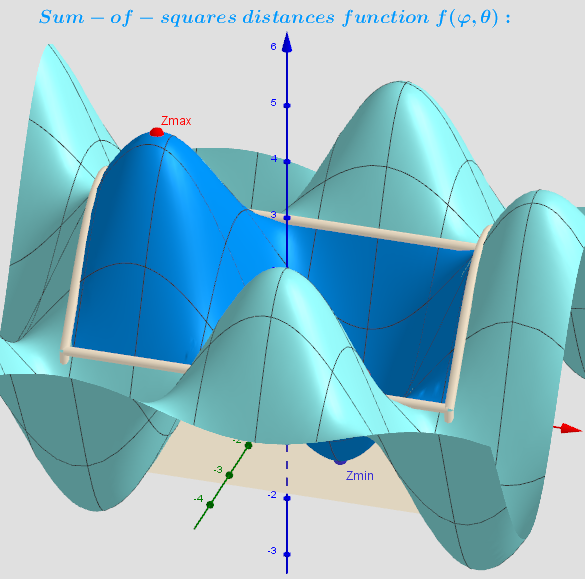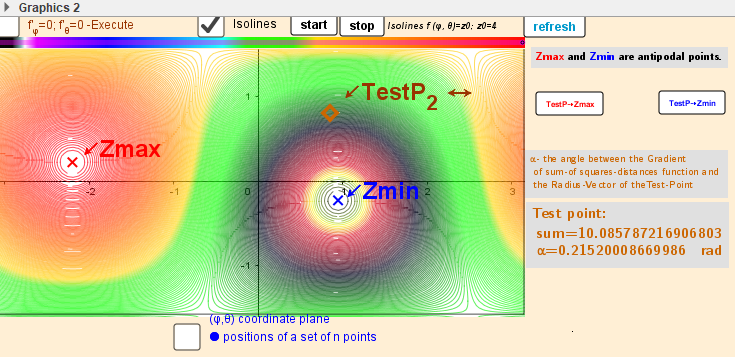Applet. Geometric centers on a sphere "induced" by moving points in three-dimensional space.

This applet is used to study the distribution of geometric centers on a sphere of radius R, „induces“ by the discrete sample of movable points in the 3-D space. Description in https://www.geogebra.org/m/nge6gawt-Settings plane, equalities from the Steiner theorem -Two-variable function f(φ,θ) over a rectangular region: - π ≤φ ≤ π; -π/2≤θ≤π/2. - Intersection Implicit Curves f'φ(φ, θ)=0; f'θ(φ,θ)=0 over a rectangular region φ∈[-π,π], θ∈[-0.5π,0.5π] -Distribution of points Pi and their local maxima/minima and saddle -critical points of distance sum function f(φ,θ) on a sphere + test Point. Vectors ∇f and ∇g at these points.Distribution of points Pi, Cm, GCmax and GCmin on a sphere. Vectors ∇f and ∇g at these points.Two-variable function f(φ,θ) over a rectangular region: - π ≤φ ≤ π; -π/2≤θ≤π/2.Intersection Implicit Curves f'φ(φ, θ)=0; f'θ(φ,θ)=0 over a rectangular region φ∈[-π,π], θ∈[-0.5π,0.5π]